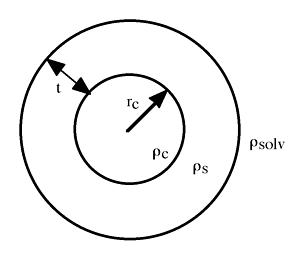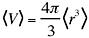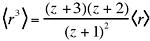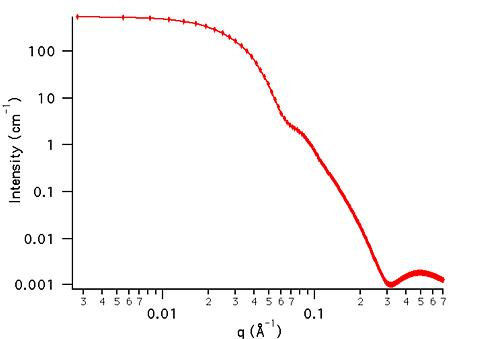MODEL

PolyCoreShell

AUTHOR/MODIFICATION

Steve Kline 06 NOV 1998

Alan Munter 08 JULY 1999, converted to Java

APPROVED FOR DISTRIBUTION

DESCRIPTION

Calculates the form factor for polydispersed spherical particles with a core-shell structure. The spherical particles have a polydisperse core with a constant shell thickness. The form factor is normalized by the average particle volume such that

P(q) = scale*<f*f>/Vol + bkg, where f is the single particle scattering amplitude, appropriately averaged over the Schulz distribution of radii.

Resolution smeared version is also provided.

VARIABLES

Input Variables (default values):

Parameter Variable Value
0Scale1.0
2Core Polydispersity (0-1)0.2
3Shell Thickness (Å)10.0
4Core SLD (Å-2)1.0e-6
5Shell SLD (Å-2)2.0e-6
6Solvent SLD (Å-2)3.0e-6
7incoherent Background(cm-1)0.000

USAGE NOTES

The returned value is scaled to units of [cm-1], on absolute scale.Parameter (scale) is correlated with the SLD's that describe the particle. No more than one of these parameters can be free during model fitting.

Parameter (polydispersity) is constrained to keep it within its physical limits of (0,1). If a value larger than 1 is entered that value is silently converted to 1 for the calculation. Polydispersity, p = s/Rc, where s2 is the variance of the distribution and Rc is the mean core radius, Parameter. For a more complete description of the Schulz distribution, see: J. Hayter in "Physics of Amphiphiles - Micelles, Vesicles and Microemulsions" V. DeGiorgio and M. Corti, Eds. (1983) p. 69.

The returned form factor is normalized by the average particle volume <V>:whereand z is the width parameter of the Schulz distribution,.

Setting the SLD of the core and shell equal to simulate a uniform sphere results in a mathematical (divide by zero) error, so if this occurs the difference is silently made equal to 10-10. To simulate a uniform SLD sphere, set the shell thickness to zero (the SLD of the shell will now make no contribution to the scattering).

The average particle diameter is 2*(Radius + shell thickness)

If the scale factor Parameter is set equal to the particle volume fraction, phi, the returned value is the scattered intensity per unit volume, I(q) = phi*P(q).

No interparticle interference effects are included in this calculation.

This function is useful for vesicles or liposomes by setting the SLD (core) = SLD (solvent), thus calculating scattering from a spherical shell with constant thickness, but polydisperse inner radius.

REFERENCE

Bartlett, P.; Ottewill, R. H. J. Chem. Phys., 1992, 96, 3306.

TEST DATASET

This example dataset is produced by calculating the PolyCoreShell using 256 data points, qmin = 0.001 Å-1, qmax = 0.7 Å-1 and the above default parameter values.